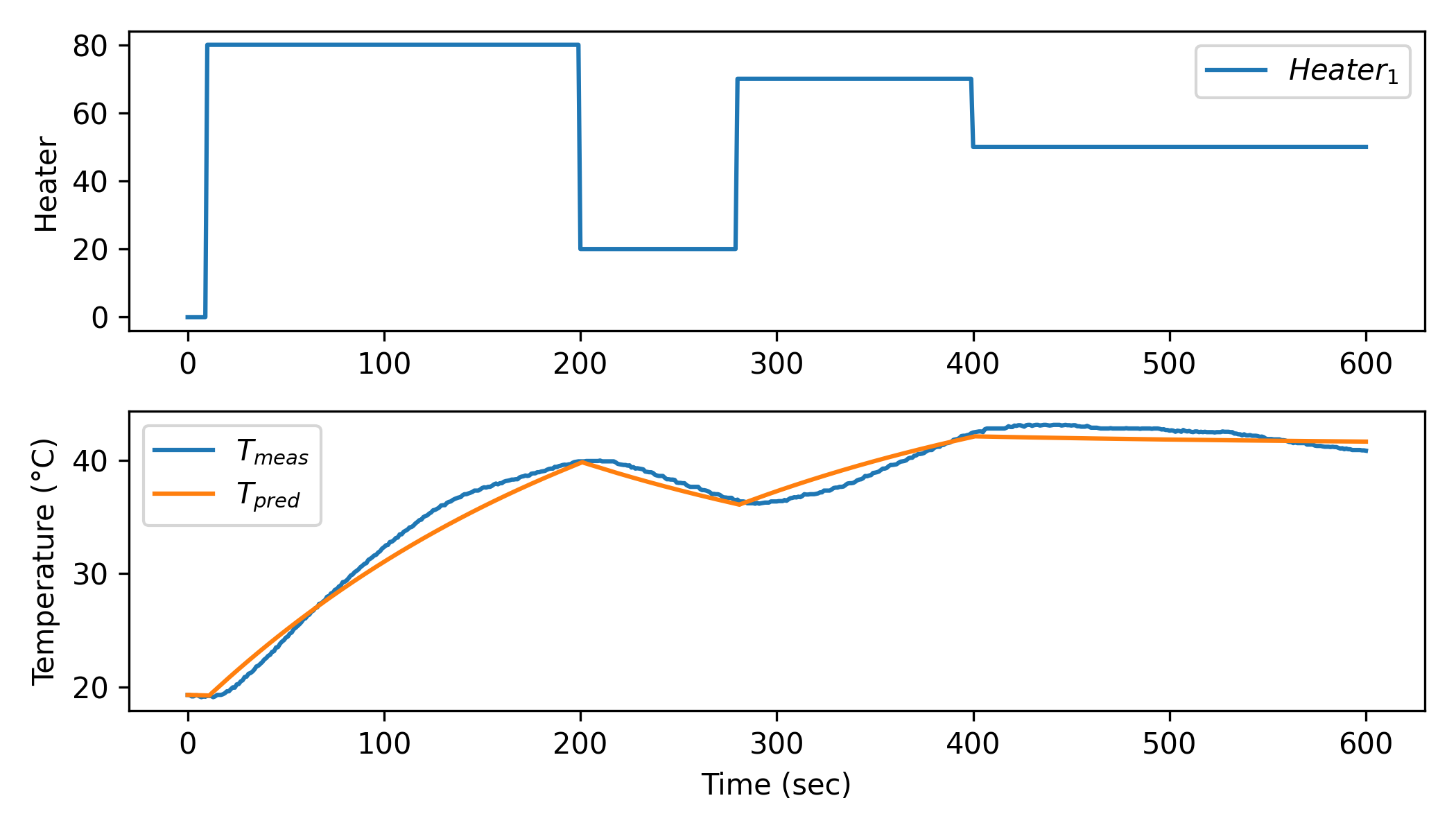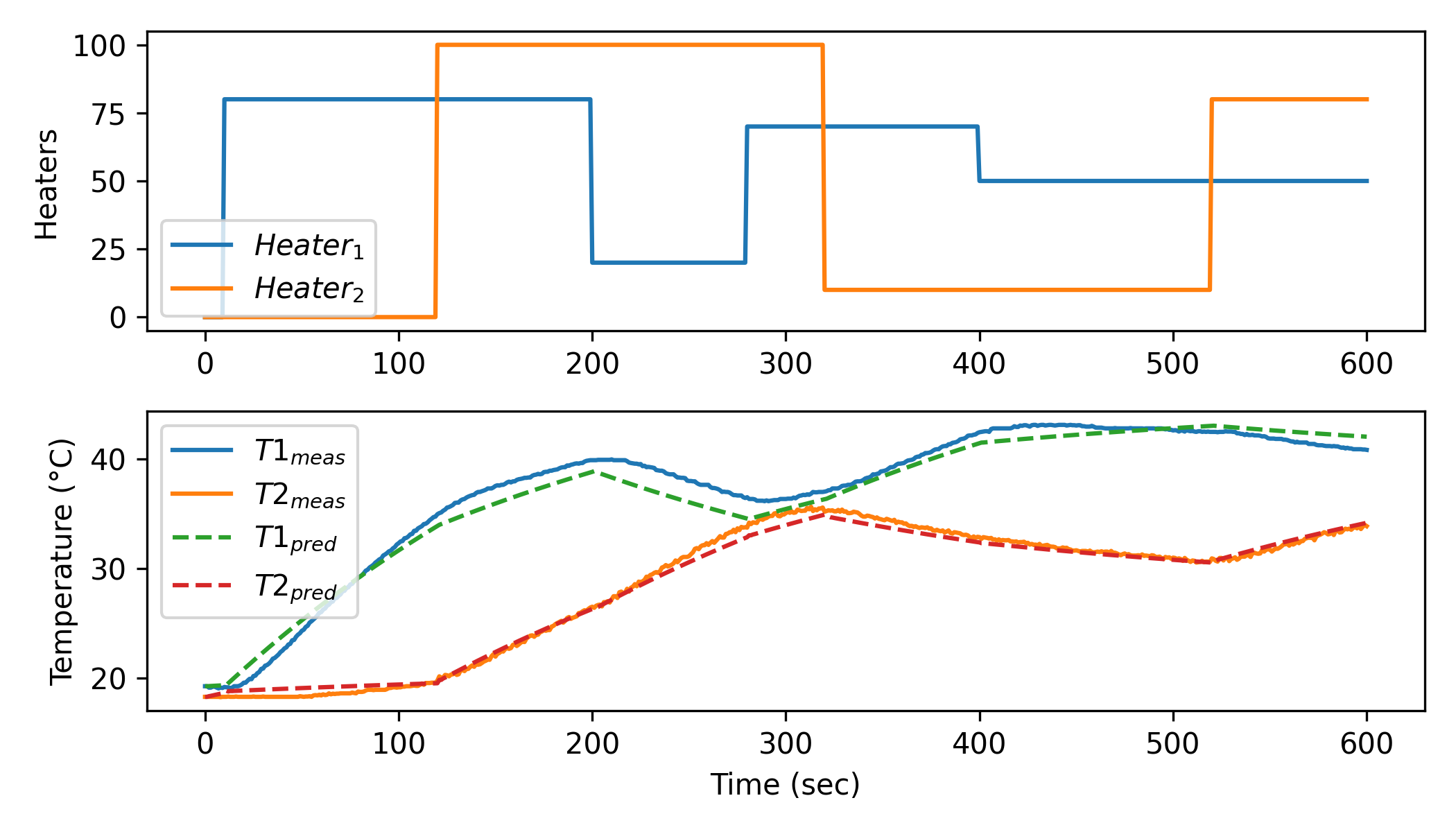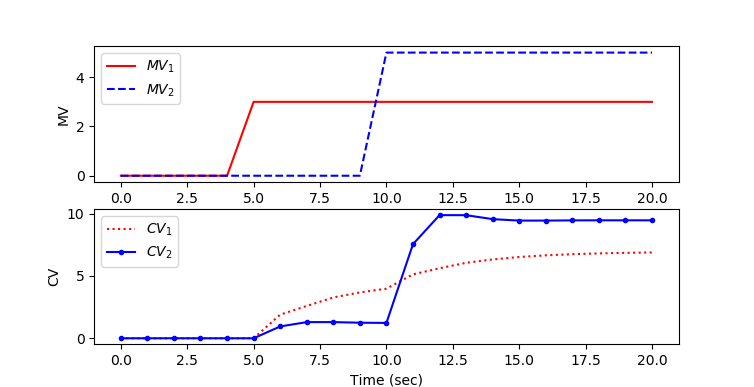### ARX Time Series ModelAPMonitor Objects
 Type: Object
Data: A, B matrices
Inputs: Input (u)
Outputs: Output (y)
Description: ARX Time Series Model
APMonitor Usage: sys = arx
GEKKO Usage: y,u = m.arx(p,y=[],u=[])


ARX time series models are a linear representation of a dynamic system in discrete time. Putting a model into ARX form is the basis for many methods in process dynamics and control analysis. Below is the time series model with a single input and single output with k as an index that refers to the time step.

$$y_{k+1} = \sum_{i=1}^{n_a} a_i y_{k-i+1} + \sum_{i=1}^{n_b} b_i u_{k-i+1}$$

With na=3, nb=2, nu=1, and ny=1 the time series model is:

$$y_{k+1} = a_1 \, y_k + a_2 \, y_{k-1} + a_3 \, y_{k-2} + b_1 \, u_k + b_2 \, u_{k-1}$$

There may also be multiple inputs and multiple outputs such as when na=1, nb=1, nu=2, and ny=2.

$$y1_{k+1} = a_{1,1} \, y1_k + b_{1,1} \, u1_k + b_{1,2} \, u2_k$$

$$y2_{k+1} = a_{1,2} \, y2_k + b_{2,1} \, u1_k + b_{2,2} \, u2_k$$

Time series models are used for identification and advanced control. It has been in use in the process industries such as chemical plants and oil refineries since the 1980s. Model predictive controllers rely on dynamic models of the process, most often linear empirical models obtained by system identification.# see https://apmonitor.com/wiki/index.php/Apps/ARXTimeSeries
from gekko import GEKKO
import numpy as np
import pandas as pd
import matplotlib.pyplot as plt

# load data and parse into columns
t = data['Time']
u = data['H1']
y = data['T1']

m = GEKKO()

# system identification
na = 2 # output coefficients
nb = 2 # input coefficients
yp,p,K = m.sysid(t,u,y,na,nb,pred='meas')

plt.figure()
plt.subplot(2,1,1)
plt.plot(t,u,label=r'$Heater_1$')
plt.legend()
plt.ylabel('Heater')
plt.subplot(2,1,2)
plt.plot(t,y)
plt.plot(t,yp)
plt.legend([r'$T_{meas}$',r'$T_{pred}$'])
plt.ylabel('Temperature (°C)')
plt.xlabel('Time (sec)')
plt.show()from gekko import GEKKO
import numpy as np
import pandas as pd
import matplotlib.pyplot as plt

# load data and parse into columns
t = data['Time']
u = data[['H1','H2']]
y = data[['T1','T2']]

m = GEKKO()

# system identification
na = 2 # output coefficients
nb = 2 # input coefficients
yp,p,K = m.sysid(t,u,y,na,nb,pred='meas')

plt.figure()
plt.subplot(2,1,1)
plt.plot(t,u,label=r'$Heater_1$')
plt.legend([r'$Heater_1$',r'$Heater_2$'])
plt.ylabel('Heaters')
plt.subplot(2,1,2)
plt.plot(t,y)
plt.plot(t,yp,'--')
plt.legend([r'$T1_{meas}$',r'$T2_{meas}$',\
r'$T1_{pred}$',r'$T2_{pred}$'])
plt.ylabel('Temperature (°C)')
plt.xlabel('Time (sec)')
plt.show()

These models are typically in the finite impulse response, time series, or linear state space form. Once in APMonitor form, nonlinear elements can be added to avoid multiple model switching, gain scheduling, or other ad hoc measures commonly employed because of linear MPC restrictions.

#### Example Model Predictive Control in GEKKOimport numpy as np
from gekko import GEKKO
import matplotlib.pyplot as plt

na = 2 # Number of A coefficients
nb = 1 # Number of B coefficients
ny = 2 # Number of outputs
nu = 2 # Number of inputs

# A (na x ny)
A = np.array([[0.36788,0.36788],\
[0.223,-0.136]])
# B (ny x (nb x nu))
B1 = np.array([0.63212,0.18964]).T
B2 = np.array([0.31606,1.26420]).T
B = np.array([[B1],[B2]])

C = np.array([0,0])

# create parameter dictionary
# parameter dictionary p['a'], p['b'], p['c']
# a (coefficients for a polynomial, na x ny)
# b (coefficients for b polynomial, ny x (nb x nu))
# c (coefficients for output bias, ny)
p = {'a':A,'b':B,'c':C}

# Create GEKKO model
m = GEKKO(remote=False)

# Build GEKKO ARX model
y,u = m.arx(p)

tf = 20 # final time
u1 = np.zeros(tf+1)
u2 = u1.copy()
u1[5:] = 3.0
u2[10:] = 5.0
u.value = u1
u.value = u2

# customize names
mv1 = u
mv2 = u
cv1 = y
cv2 = y

# options
m.time = np.linspace(0,tf,tf+1)
m.options.imode = 4
m.options.nodes = 2

# simulate
m.solve()

plt.figure(1)
plt.subplot(2,1,1)
plt.plot(m.time,mv1.value,'r-',label=r'$MV_1$')
plt.plot(m.time,mv2.value,'b--',label=r'$MV_2$')
plt.ylabel('MV')
plt.legend(loc='best')
plt.subplot(2,1,2)
plt.plot(m.time,cv1.value,'r:',label=r'$CV_1$')
plt.plot(m.time,cv2.value,'b.-',label=r'$CV_2$')
plt.ylabel('CV')
plt.xlabel('Time (sec)')
plt.legend(loc='best')
plt.show()

#### Example Model in APMonitor

 Objects
sys = arx
End Objects

Parameters
mv1
mv2
End Parameters

Variables
cv1 = 0
cv2 = 0
End Variables

Connections
mv1 = sys.u
mv2 = sys.u
cv1 = sys.y
cv2 = sys.y
End Connections

File sys.txt
2 ! inputs
2 ! outputs
1 ! number of input terms
2 ! number of output terms
End File

File sys.alpha.txt
0.36788, 0.36788
0.223, -0.136
End File

File sys.beta.txt
0.63212, 0.18964
0.31606, 1.2642
End File


Also see: